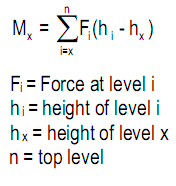## Tuesday, December 2, 2014

### Wind effect - Structural Design

A building in the path of wind causes wind pressure which in turn causes force, shear, and overturn moment at each level that must be resisted, following a load path to the foundations (wind wall pressure transfers to horizontal diaphragms, then to shear walls, finally to foundation).  Wind pressure times tributary area per level causes  lateral force per level.  Shear per level, the sum of wind forces above, defines required resistance.   Overturn moment per level is the sum of forces above times their height above the respective level.

1  Wind force, shear, and overturn moment per level

Fx = wind force = wind pressure times tributary area per level exposed to wind
VX = shear per level = sum of Fx  above
Mx = overturn moment  = sum of all forces above times their distance above level x.

2 Overturn effect

3  Windward  pressure increase with height

4 Wind force

Fx = (windward pressure + leeward suction) times tributary area per level
(leeward wind suction is assumed constant for full height)
Fx = P A
P = wind pressure and suction in psf (Pa)
A = tributary area exposed to wind
(tributary area = building width times half the story height above and below)

5 Shear

Wind shear per level = sum of all wind forces above
Wind shear is the integration of wind forces above

6 Overturn moment

Overturn moment per level  = sum of all forces above times their distance
Overturn moment per level = integration of shear diagram above respective level